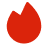# IBM计算机科学面试真题

BQ

Coding（0）
System Design（0）
Algorithm（6）
Ood（0）高频题（0）

Coding（0）
System Design（0）
Algorithm（6）
Ood（0）高频题（0）
1.LeetCode 221
2.LeetCode 211
3.LeetCode 1560
4.LeetCode 134
5.LeetCode 844
6.Valid subsequence
7.Sentence partation
8.聊天机器人
1. LeetCode 221
Given an m x n binary matrix filled with 0's and 1's, find the largest square containing only 1's and return its area.

Example 1:

```Input: matrix = [["1","0","1","0","0"],["1","0","1","1","1"],["1","1","1","1","1"],["1","0","0","1","0"]]
Output: 4
```
Example 2:

```Input: matrix = [["0","1"],["1","0"]]
Output: 1
```
Example 3:

```Input: matrix = [["0"]]
Output: 0
```

Constraints:

• m == matrix.length
• n == matrix[i].length
• 1 <= m, n <= 300
• matrix[i][j] is '0' or '1'.
2. LeetCode 211
Design a data structure that supports adding new words and finding if a string matches any previously added string.

Implement the WordDictionary class:

• WordDictionary() Initializes the object.
• void addWord(word) Adds word to the data structure, it can be matched later.
• bool search(word) Returns true if there is any string in the data structure that matches word or false otherwise. word may contain dots '.' where dots can be matched with any letter.

Example:

```Input
Output
[null,null,null,null,false,true,true,true]

Explanation
WordDictionary wordDictionary = new WordDictionary();
wordDictionary.search("pad"); // return False
wordDictionary.search("bad"); // return True
wordDictionary.search(".ad"); // return True
wordDictionary.search("b.."); // return True
```

Constraints:

• 1 <= word.length <= 25
• word in addWord consists of lowercase English letters.
• word in search consist of '.' or lowercase English letters.
• There will be at most 3 dots in word for search queries.
• At most 104 calls will be made to addWord and search.
3. LeetCode 1560
Given an integer n and an integer array rounds. We have a circular track which consists of n sectors labeled from 1 to n. A marathon will be held on this track, the marathon consists of m rounds. The ith round starts at sector rounds[i - 1] and ends at sector rounds[i]. For example, round 1 starts at sector rounds and ends at sector rounds

Return an array of the most visited sectors sorted in ascending order.

Notice that you circulate the track in ascending order of sector numbers in the counter-clockwise direction (See the first example).

Example 1:

```Input: n = 4, rounds = [1,3,1,2]
Output: [1,2]
Explanation: The marathon starts at sector 1. The order of the visited sectors is as follows:
1 --> 2 --> 3 (end of round 1) --> 4 --> 1 (end of round 2) --> 2 (end of round 3 and the marathon)
We can see that both sectors 1 and 2 are visited twice and they are the most visited sectors. Sectors 3 and 4 are visited only once.```
Example 2:

```Input: n = 2, rounds = [2,1,2,1,2,1,2,1,2]
Output: 
```
Example 3:

```Input: n = 7, rounds = [1,3,5,7]
Output: [1,2,3,4,5,6,7]
```

Constraints:

• 2 <= n <= 100
• 1 <= m <= 100
• rounds.length == m + 1
• 1 <= rounds[i] <= n
• rounds[i] != rounds[i + 1] for 0 <= i < m
4. LeetCode 134
There are n gas stations along a circular route, where the amount of gas at the ith station is gas[i].

You have a car with an unlimited gas tank and it costs cost[i] of gas to travel from the ith station to its next (i + 1)th station. You begin the journey with an empty tank at one of the gas stations.

Given two integer arrays gas and cost, return the starting gas station's index if you can travel around the circuit once in the clockwise direction, otherwise return -1. If there exists a solution, it is guaranteed to be unique

Example 1:

```Input: gas = [1,2,3,4,5], cost = [3,4,5,1,2]
Output: 3
Explanation:
Start at station 3 (index 3) and fill up with 4 unit of gas. Your tank = 0 + 4 = 4
Travel to station 4. Your tank = 4 - 1 + 5 = 8
Travel to station 0. Your tank = 8 - 2 + 1 = 7
Travel to station 1. Your tank = 7 - 3 + 2 = 6
Travel to station 2. Your tank = 6 - 4 + 3 = 5
Travel to station 3. The cost is 5. Your gas is just enough to travel back to station 3.
Therefore, return 3 as the starting index.
```
Example 2:

```Input: gas = [2,3,4], cost = [3,4,3]
Output: -1
Explanation:
You can't start at station 0 or 1, as there is not enough gas to travel to the next station.
Let's start at station 2 and fill up with 4 unit of gas. Your tank = 0 + 4 = 4
Travel to station 0. Your tank = 4 - 3 + 2 = 3
Travel to station 1. Your tank = 3 - 3 + 3 = 3
You cannot travel back to station 2, as it requires 4 unit of gas but you only have 3.
Therefore, you can't travel around the circuit once no matter where you start.
```

Constraints:

• n == gas.length == cost.length
• 1 <= n <= 105
• 0 <= gas[i], cost[i] <= 104
5. LeetCode 844
Given two strings s and t, return true if they are equal when both are typed into empty text editors. '#' means a backspace character.

Note that after backspacing an empty text, the text will continue empty.

Example 1:

```Input: s = "ab#c", t = "ad#c"
Output: true
Explanation: Both s and t become "ac".
```
Example 2:

```Input: s = "ab##", t = "c#d#"
Output: true
Explanation: Both s and t become "".
```
Example 3:

```Input: s = "a#c", t = "b"
Output: false
Explanation: s becomes "c" while t becomes "b".
```

Constraints:

• 1 <= s.length, t.length <= 200
• s and t only contain lowercase letters and '#' characters.

Follow up: Can you solve it in O(n) time and O(1) space?

6. Valid subsequence

Follow-up：如果有很多个B，要对同一个A做判断，该怎么优化？
7. Sentence partation

8. 聊天机器人